Math Vocabulary
Multiplication
Graphs
100

Solve the problem:

24+31=

55

100

Solve the problem:

58-43=

15

100

What do you call the two numbers that are being added together?

100

Solve the problem:

3x0=

0

100

What does a bar graph use to show data?

Bars

200
What is the value of a nickel?

5 cents

200

Solve the problem:

100-68=

32

200

Hundred, ten, and ones are examples of what?

place value

200

True or False:

If you switch the factors in a multiplication problem you get a different answer.

False

You can change the numbers and get the same answer. This is the commutative property.

200

How many students picked green as their favorite color?4 students

300

Write 643 in expanded form

600+40+3
300

Give an an example of a whole number.

300

What do you call the answer in multiplication?

Product
300

Write an equation for this array and solve: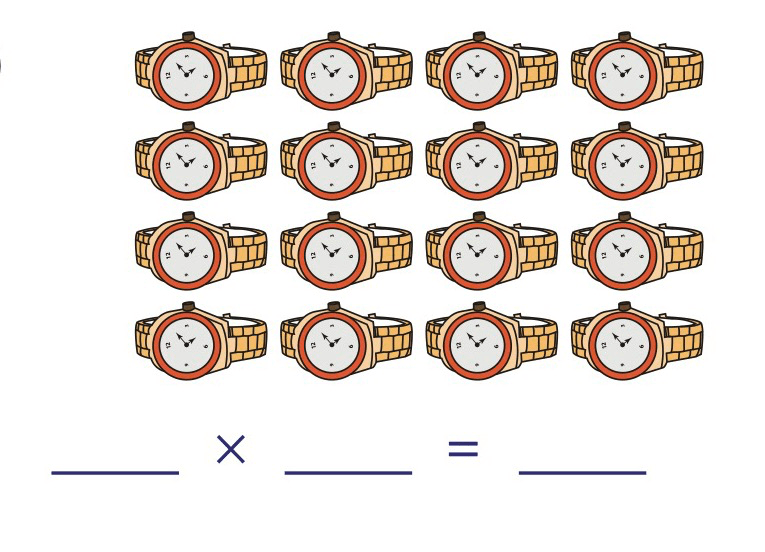4x4=16

300

How many fewer students picked red than green?3 children

400

Solve:

514

-319

_________

195

400

Write the fraction: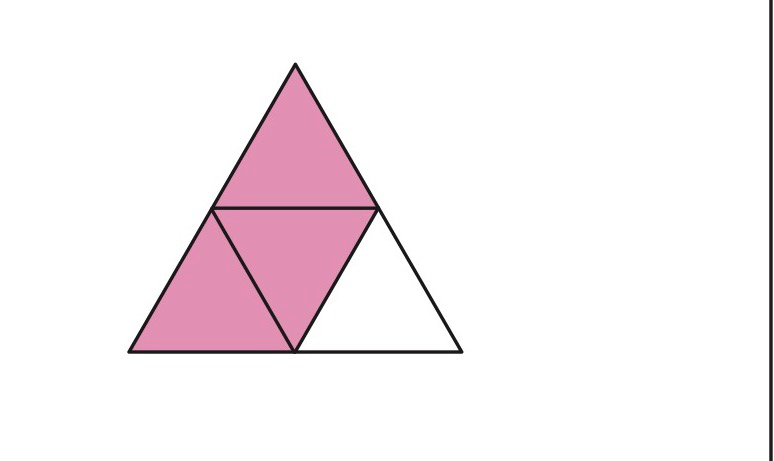3/4

400

In the fraction 2/3 , what do 2 and 3 represent?

2 is the numerator and 3 is the denominator

400

Name two strategies you can use when solving multiplication.

400

A. How many fewer students picked Reading than Math?

B. Which of these are true? Choose the two correct answers.

1. There were 10 students who picked writing or math.

2. There was 1 fewer student who picked Science than Reading

3. Most popular subject was Math.

4. More students chose Science than writing.

A. 4 studnets

B.

1. There were 10 students who picked writing or math.

2. Most popular subject was Math

500

Measure the object.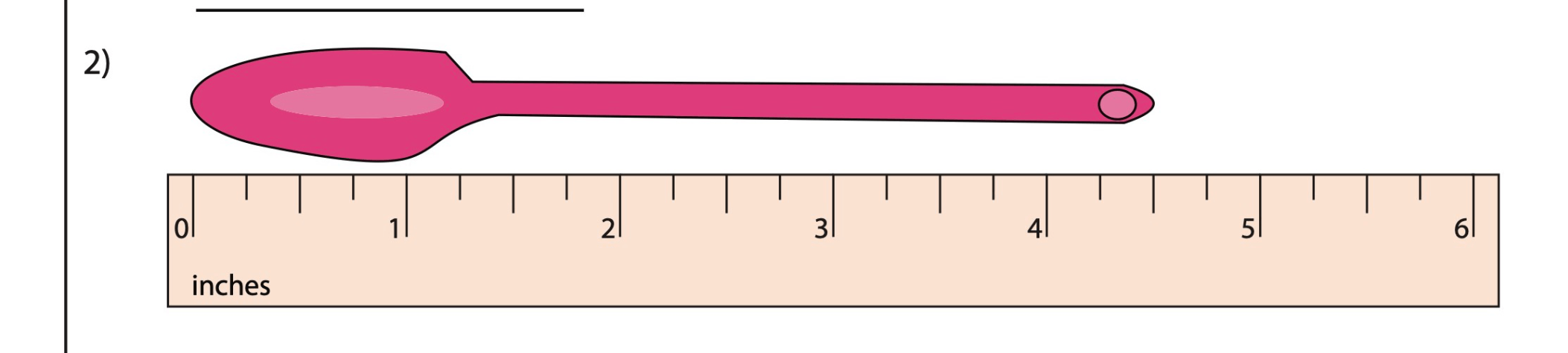4 1/2 inches

500

Calculate how much many each side has?

Which side has the greater amount?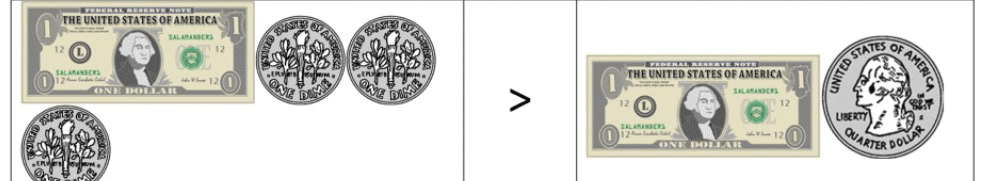\$1.30> \$1.25

500

true or false:

An octagon has 6 sides.

False. 8 sides

500

Write and solve the equation for this model: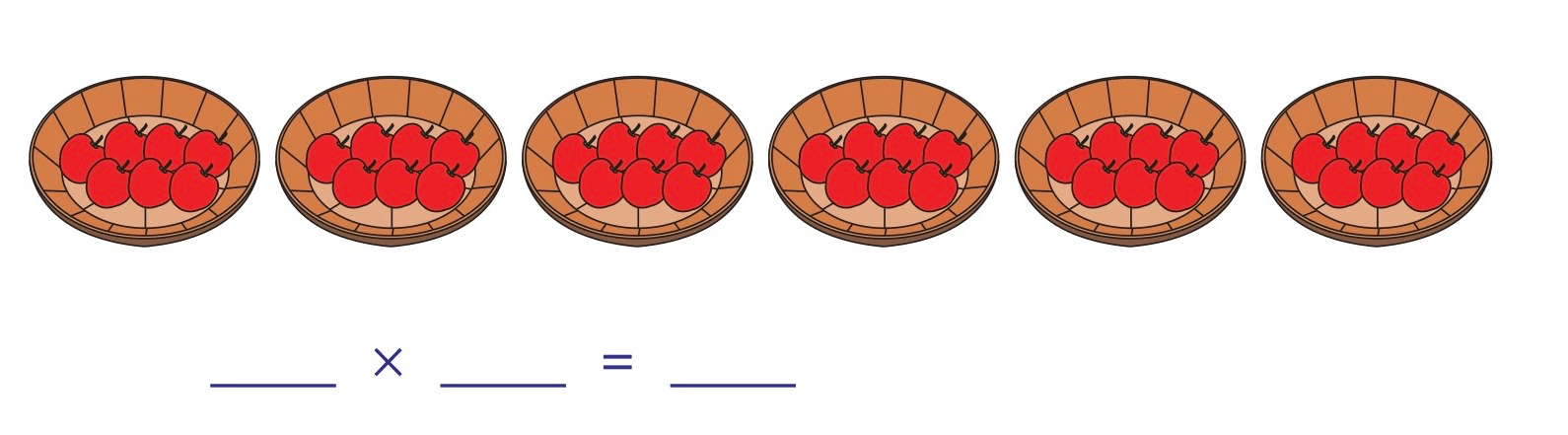6x7=42

500

Use the following data to draw a bar graph.

Number of points:

Mark- 4 points

Lily- 3 points

Dave- 5 points

Maria- 1 point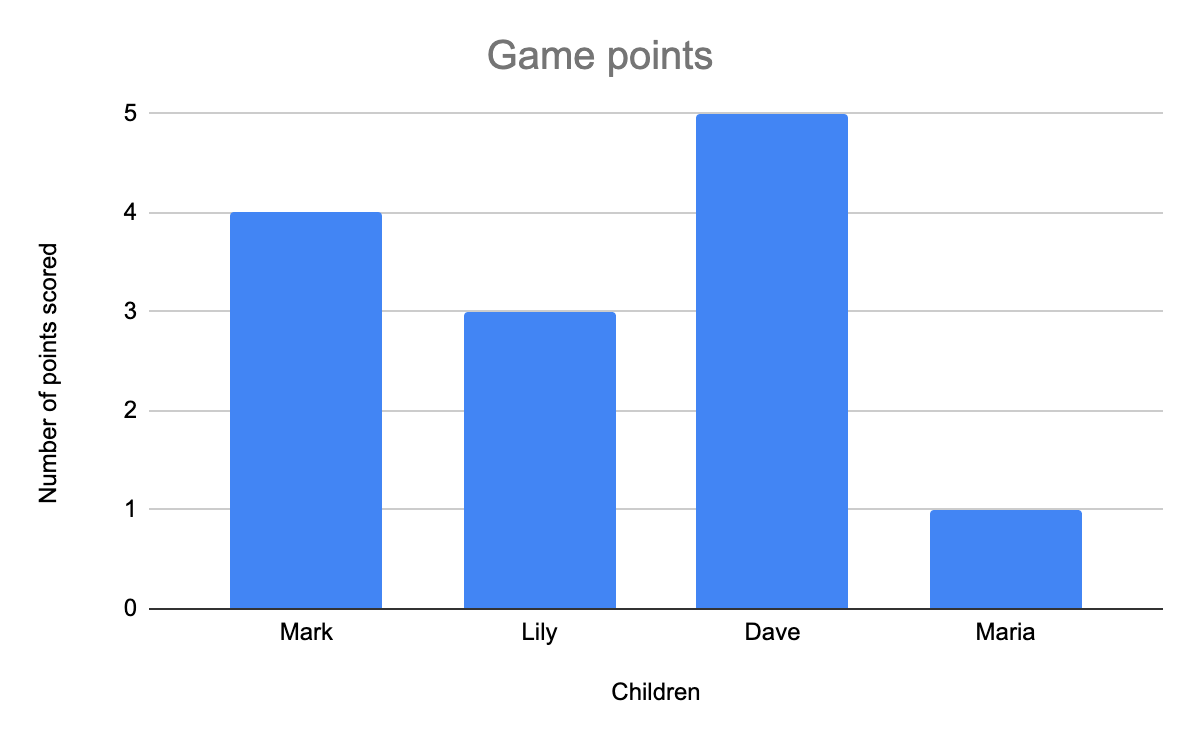Click to zoom